Solving One Way System (Alternative No. 1):
The following Slab is to be modeled using Etabs (figure 1), taking in consideration using B300 in defining both slabs and beams.

Beams Sections: B70x25 (70cm width, 25cm depth).
Slab section: S25 (25cm solid slab)
Concrete material: B300[Fig.1]

1- Start a new model (do not forget to set units before starting a new model).
2- Draw the suitable grid using Etabs.
3- Define B300 (see figure 2).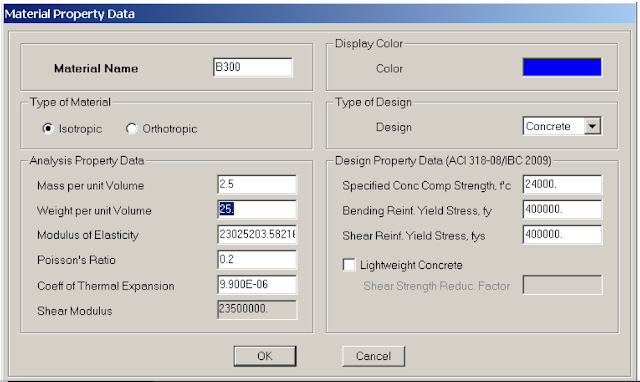[Fig.2]

4- Define B30x25 and B70x25 (see figure 3).[Fig.3]

5- Define the slab section (see figure4).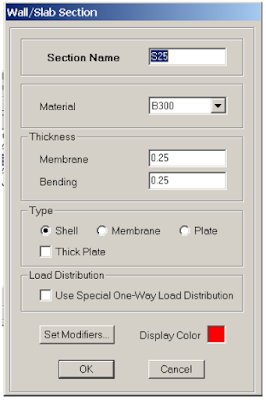[Fig.4]

6- Draw Beams in both directions (user may draw beams as one line, or as segments; if beams is drawn as a one beam, user may use divide option to divide lines into segments).
7- Draw the Slab so you can have as shown previously in figure 1. To Show sections names select the check icon(see figure 5 now).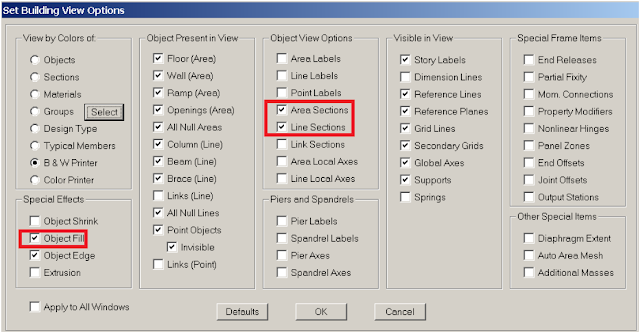[Fig.5]

8- To assign dead load to slab, first select the slab (now do as shown in figure 6).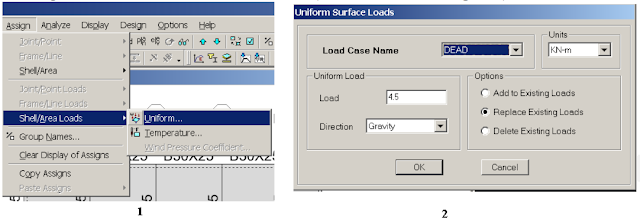[Fig.6]

9- Now assign live load, first reselect the slab again (see figure 7) (user may use the option: previous selectionto reselect the slab again).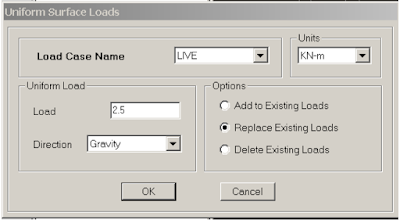[Fig.7]

10- Define the load combinations (see figure 8).[Fig.8]

11- User now must mesh the slab (mesh means dividing the slab into smaller areas). First select the slab (now see figure 9).[Fig.9]

12- To this moment, slab will act as a two way slabs; loads will be distributed to beams at both directions. To make slab action one way first select the slab (now do as shown in figure 10).[Fig.10]

13- Assign pin supports at each beam intersection (see figure 11).[Fig.11]

14- Before having analysis done user must check the model (do as shown in figure 12).[Fig.12]

15- Now start Analysis (hit F5) or do as shown in figure 13.[Fig.13]

16- User may check both deflection shape and moment results to make sure slab works as a one way slab (see figure 14).[Fig.14]